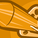# New to Qlik Sense

If you’re new to Qlik Sense, start with this Discussion Board and get up-to-speed quickly.

Announcements
cancel
Showing results for
Did you mean:Creator III

## Suppressing Null in Measure

Hi All,

I have the following Measure.

Only(
{<[Send Date]={">=\$(=Weekstart(WeekName(max([Send Date]),-4)))<=\$(=Weekend(WeekName(max([Send Date]))))"}>}

[Purchase Order No])

I want to only show the Purchase Order No for the last 4 weeks. This is working however, i have Null Values for the Purchase Order No where not in the last 4 weeks.

I Have not been able to make this measure into an expression with an If statement to suppress the null values and i have found the Add ons option is not working.

How can i make the above measure as a dimension please so i can just suppress the null values.

Thank you

Daniel

Labels (2)

• ### Set Analysis

1 Solution

Accepted SolutionsChampion III

You have two options,

Option 1, move the conditions to a measure instead of dimension

example

Dimension = [Purchase Order No]

MEasure = count({<[Send Date]={">=\$(=Weekstart(WeekName(max([Send Date]),-4)))<=\$(=Weekend(WeekName(max([Send Date]))))"}>} [Purchase Order No])

Option Two, use a calculated dimension

=Aggr(only({<[Send Date]={">=\$(=Weekstart(WeekName(max([Send Date]),-4)))<=\$(=Weekend(WeekName(max([Send Date]))))"}>} [Purchase Order No]),[Purchase Order No]) <<-- uncheck show null values for this dimension

Option 1 is the most sensible option to go with; you want to aggregate data where sendate is within last 4 weeks, your dimensions will also get filtered based on your measures scope of calculations

however if your chart is not going to have any measures then using calculated dimension is the only option

Vineeth Pujari
If a post helps to resolve your issue, please accept it as a Solution.
2 RepliesChampion III

You have two options,

Option 1, move the conditions to a measure instead of dimension

example

Dimension = [Purchase Order No]

MEasure = count({<[Send Date]={">=\$(=Weekstart(WeekName(max([Send Date]),-4)))<=\$(=Weekend(WeekName(max([Send Date]))))"}>} [Purchase Order No])

Option Two, use a calculated dimension

=Aggr(only({<[Send Date]={">=\$(=Weekstart(WeekName(max([Send Date]),-4)))<=\$(=Weekend(WeekName(max([Send Date]))))"}>} [Purchase Order No]),[Purchase Order No]) <<-- uncheck show null values for this dimension

Option 1 is the most sensible option to go with; you want to aggregate data where sendate is within last 4 weeks, your dimensions will also get filtered based on your measures scope of calculations

however if your chart is not going to have any measures then using calculated dimension is the only option

Vineeth Pujari
If a post helps to resolve your issue, please accept it as a Solution.Creator III
Author

Thank you very much for your help on this, used option 2 and it worked as a dimension perfectly.

Daniel